# How to Count How Many Times a Word Appears in Excel (Easy Formulas)

One common requirement when working with large datasets is counting how many times a particular word or value appears.

You may need to know how many times the word appears in a cell, a column, or an entire worksheet.

This problem can be of two types. You might need to count how many cells contain a given word, or how many times a given word appears in a cell.

In this tutorial, we will see both types of problems and different ways to solve them, including the use of VBA.

## Count a Specific Word in a Range using COUNTIF

The COUNTIF function’s main task is to count the number of times a condition is met.

One of the most common uses of this function is to match a particular value to cell values in a range. This value can be a string or a number.

The syntax for the COUNTIF function is:

`COUNTIF(range,condition)`

Here,

• range is the range of cells you want to match and count from
• condition is the condition that must be met in order to count a cell as a match

What the above function will do is search for all the cells in the given range and count all the cells that match the given condition. Once it is done searching through all cells in the range, it will return the number of matched cells.

Let us apply this function to a simple example. Say we want to count the number of times the name ‘Peter’ appears in the range A2:A10 as shown below: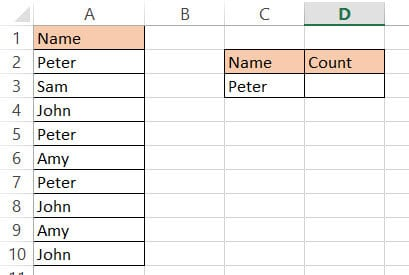To apply the COUNTIF function for the above problem, here are the steps to follow:

1. Select the cell that you want to write the count in (cell D3 in our case).
2. In this cell, type the formula:
` =COUNTIF(A2:A10,"Peter")`
3. Press the return key.

This will give the number of times the word “Peter” appears in the range of cells A2:A10.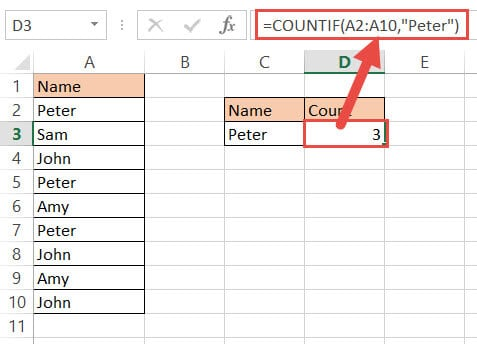Note: This method only counts the number of cells that contain exactly the word “Peter”. If one of the cells, for example, had the words “Peter Peter” in one cell, the COUNTIF function would not consider it as a match, and so would not count the cell.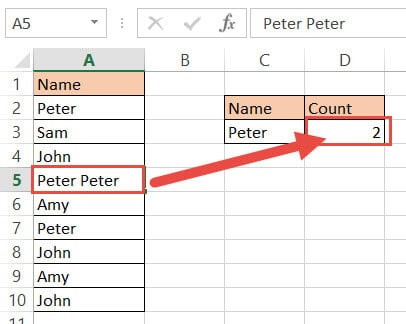## Count a Specific Word in a Cell using LEN and SUBSTITUTE

The above method works fine if you’re looking to count cells that exactly match a given word. However, it does not work if you want to find the number of times a word occurs inside the string of a cell.

For example, say you have the following string in a cell (A2) and you want to know how many times the word “happy” appears: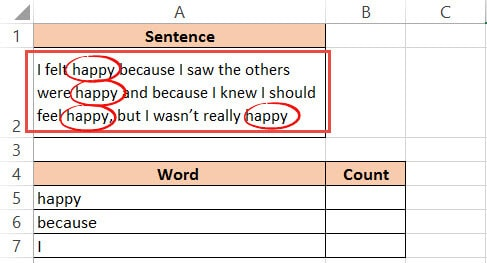To solve this problem, there are a number of methods that you can use.

For example, you can use a formula that comprises a combination of Excel functions, like SUBSTITUTE and LEN. You can also use VBA code to get the job done quickly.

### The LEN Function

The LEN function is a very commonly used Excel function. It is used to find the length of a string (number of characters in a string). The syntax for the function is:

`=LEN(string)`

Where string can be a text or reference to a cell containing text for which you want to find the length.

### The SUBSTITUTE Function

The SUBSTITUTE function is used to remove a specific word from a string. The general syntax for this function is:

`=SUBSTITUTE(original_string, old_text,new_text)`

Here,

• original_string is the text or cell reference that you want to work on.
• old_text is the word or substring that you want to replace
• new_text is the word or substring that you want to replace old_text with.

### Combining both Functions

Individually, both the above functions don’t seem to have anything to do with counting how many times a word appears in a cell. But when they are cleverly combined into a formula, they accomplish the task.

To count how many times a word appears in a cell, we can use the formula:

`=(LEN(cell_reference)-LEN(SUBSTITUTE(cell_reference,word,"")))/LEN(word)`

Here, word is the word that you want to count and cell_reference is the reference to the cell you want to count from.

Here’s how you can apply the above formula to the problem:

1. Select the cell that you want to write the count in (cell B5 in this case).
2. In this cell, type the formula:
`=(LEN(A2)-LEN(SUBSTITUTE(A2, “happy”,"")))/LEN(“happy”). Instead of specifying the word to replace, you can also specify the reference to the cell containing the word, like this: =(LEN(A2)-LEN(SUBSTITUTE(A2, A5,"")))/LEN(A5).`
3. Press the return key.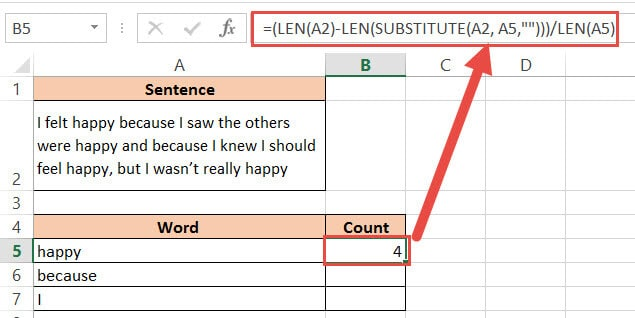This will give the number of times the word “happy” appears in cell A2.

If you also want to copy the same formula to count the number of occurrences of other words in cell A2, you can fix the reference to cell A2 by adding a \$ sign:

`=(LEN(\$A\$2)-LEN(SUBSTITUTE(\$A\$2, A5,"")))/LEN(A5)`

Now, you can easily copy the formula for other words by dragging down the fill handle.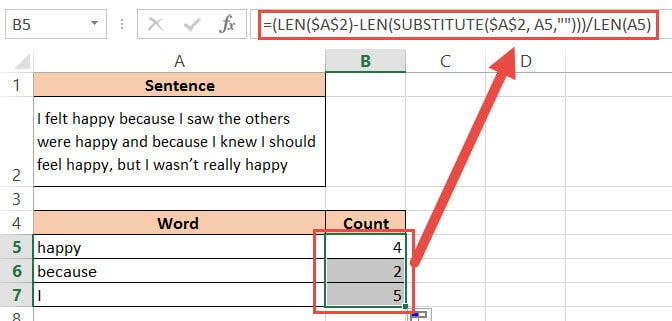### How did this Formula Work?

To understand how this formula worked, we need to break it down:

1. First, we used the SUBSTITUTE function to remove the word “happy” from the original string (by replacing it with a blank): SUBSTITUTE(A2, “happy”,””)
2. Next, we used the LEN function to find the length of the original string without any occurrence of the word “happy” in it: LEN(SUBSTITUTE(A2, “happy”,””))
3. After this, we subtracted this length from the length of the original string (with the words “happy” in it): LEN(A2)- LEN(SUBSTITUTE(A2, “happy”,””))
4. What this gives is the total number of characters in all occurrences of the word “happy”. It’s a 5 letter word, so if it is present 4 times, the above operation will return 20.
5. Finally, this value is divided by the length of the word “happy”: =(LEN(\$A\$2)-LEN(SUBSTITUTE(\$A\$2, A5,””)))/LEN(A5).

This should give you the number of times the word “happy” appears in the original text! So we get 20 / 5 = 4. The word “happy” appears 4 times in cell A2.

Note: The SUBSTITUTE function is case-sensitive. As a result, this formula will only count the number of times “happy” appears in all small letters. If you were to search for the word in all caps, you would not get the correct answer.

A good way to work around this is to ensure that everything is converted to small letters. So to make sure our formula is not case-sensitive, it can be converted to:

`=(LEN(cell_reference)-LEN(SUBSTITUTE(LOWER(cell_reference), LOWER(word),"")))/LEN(word)`

Here, the function, LOWER is used to convert both the original text and new text to lowercase.

## Count How many times a Word Appears in a Range

The above method only works when you want to find the number of times a word appears in a single cell. But what if you needed to find out how many times the same word appears in strings in multiple cells?

For this, you will need to wrap the SUMPRODUCT function around the above formula. So, if you have a range of cells to work on, your formula should be:

`=SUMPRODUCT(LEN(cell_range)-LEN(SUBSTITUTE(cell_range, word,"")))/LEN(word))`

The SUMPRODUCT function ensures that you get an array containing the count for each cell in the range.

Say you have the following set of text strings (A2:A3) and you want to find out how many times the word “happy” appears in all of them: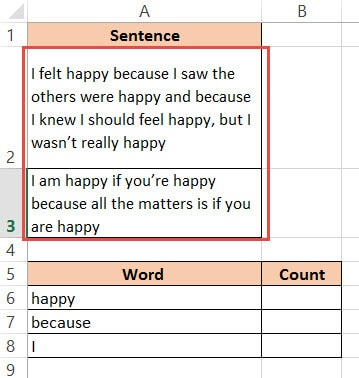1. Select the cell that you want to write the count in (cell B6 in this case).
2. In this cell, type the formula:
`=SUMPRODUCT((LEN(A2:A3)-LEN(SUBSTITUTE(A2:A3,”happy”,"")))/LEN(“happy”)). Instead of specifying the word to replace, you can also specify the reference to the cell containing the word, like this: =SUMPRODUCT((LEN(A2:A3)-LEN(SUBSTITUTE(A2:A3,A6,"")))/LEN(A6))`
3. Press the return key.

This will give the number of times the word “happy” appears in range A2:A3.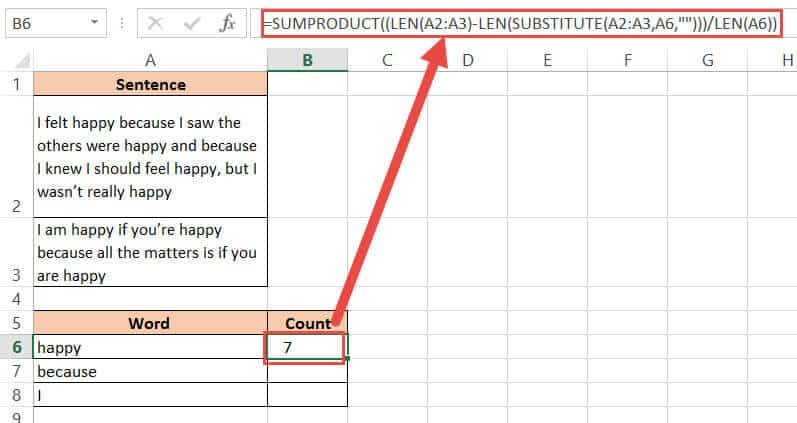If you also want to copy the same formula to count the number of occurrences of other words in the same range, you can fix the reference to the range cell A2:A3 by adding a \$ sign:

`=SUMPRODUCT((LEN(\$A\$2:\$A\$3)-LEN(SUBSTITUTE(\$A\$2:\$A\$3,A6,"")))/LEN(A6))`

Now, you can easily copy the formula for other words by dragging down the fill handle.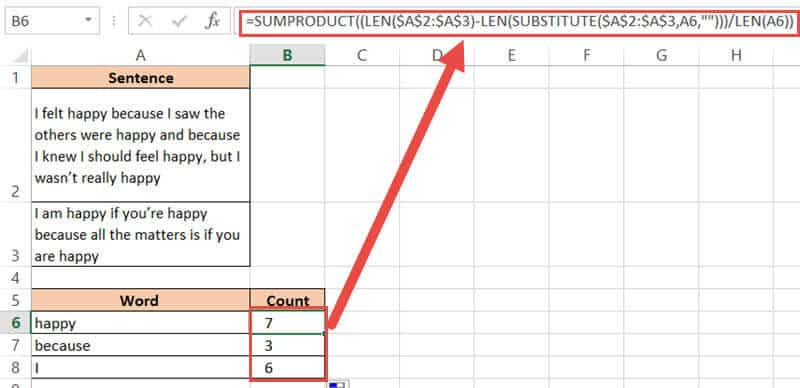## Using VBA to Count the Number of Times  a Word Appears in any Range

You can accomplish the same task as above by using VB Script.

Here’s the VBA code that we will be using to count the number of times the word “happy” appears in the code. Feel free to select and copy it.

```Sub count_word_occurrences()
Count = 0
search_word = "happy"
Dim rng As Range
Dim cell As Range
Set rng = Application.Selection
For Each cell In rng
Count = Count + ((Len(cell) - Len(Replace\$(cell, search_word, ""))) / Len(search_word))
Next cell
MsgBox ("The string " & search_word & " occurred " & Count & " times")
End Sub```

1. From the Developer Menu Ribbon, select Visual Basic.
2. Once your VBA window opens, Click Insert->Module. Now you can start coding. Type or copy-paste the above lines of code into the module window. Your code is now ready to run.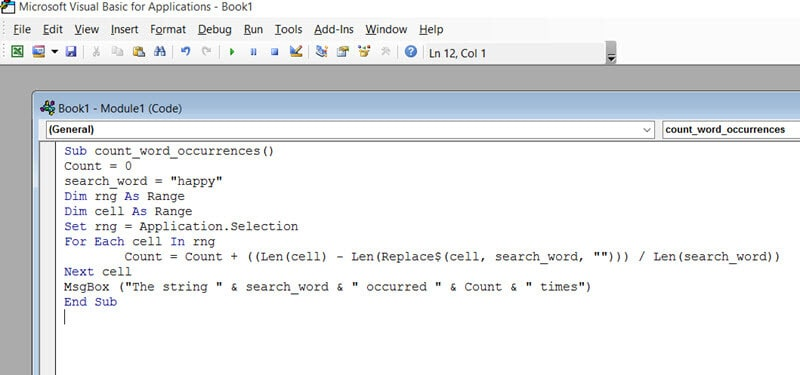3. Select a single cell or cells containing the text you want to count from.
4. Navigate to Developer->Macros->count_word_occurrences->Run.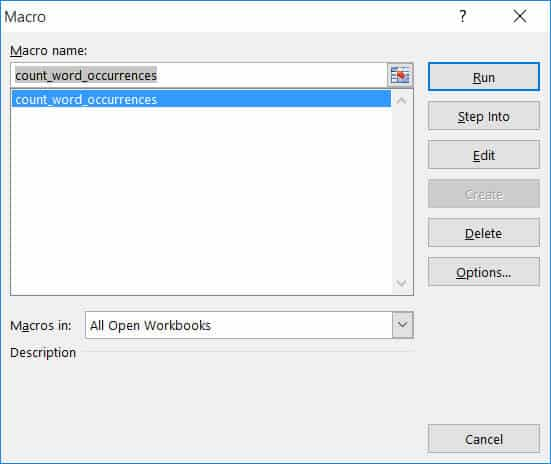5. You will now see a message box telling you how many times the word “happy” appears in your selected range.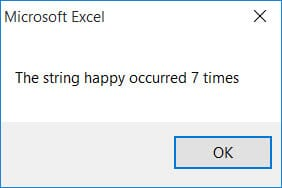Note: If you can’t see the Developer ribbon, from the File menu, go to Options. Select Customize Ribbon and check the Developer option from the Main Tabs. Finally, Click OK.

The great thing about this method is that it does not matter whether you select one cell or a range of cells. It works both ways.

Here are a few ways you can tweak the above code to suit your requirements:

• You can customize this code by changing the search_word value in line 3 to the word that you want to count. So if you want to count the number of times the word “because” appears, you can replace this line with: search_word = ”because”
• You can use a cell reference instead of specifying the actual word to search in the code itself. So, if you want to use a cell reference, say A6, instead of specifying the search word “happy”, you can replace line 3 with: search_word = Cells(6,”A”).Value
• Similarly, you can display the result in a cell instead of a message box. So, if you want to display the count in a cell, say B6, instead of displaying it in a message box, you can replace line 10 with: Cells(6,”B”).Value = Count
• If you want the search to be case-insensitive, you can use the LCase function. So, you will need to change line 8 to: Count = Count + ((Len(cell) – Len(Replace\$(LCase(cell), LCase(search_word), “”))) / Len(search_word))

In this tutorial, we demonstrated how you can count the number of times a word appears in Excel. We showed how to count the exact occurrences of a word in a range of cells using COUNTIF.

We then showed you how you can count how many times a word appears inside the string of a single cell, using SUBSTITUTE and LEN functions.

After that, we demonstrated how you can apply this to a whole range of cells containing strings, by just wrapping the same formula in a SUMPRODUCT function.

Finally, we provided a VBA code snippet to let you get this task done quickly.

We made this tutorial keeping in mind the different kinds of issues you might face when trying to count occurrences of a word in your worksheet. We do hope this was helpful.

Other Excel tutorials you may like: